Name:    Quiz 7.6-7.6

Multiple Choice
Identify the choice that best completes the statement or answers the question.

Write and solve an equation to find the missing dimension of the figure. Check your solution.

1.

Area = 54 ft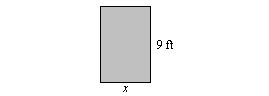a. 486 ft c. 18 ft b. 6 ft d. 7 ft

2.

Circumference = 56.52 mm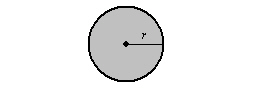a. 9 mm c. 18 mm b. 27 mm d. 177.47 mm

3.

Perimeter = 28 in.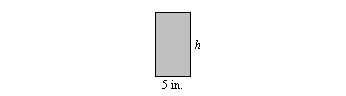a. 7 in. c. 10 in. b. 5.6 in. d. 9 in.

4.

Area = 42 cm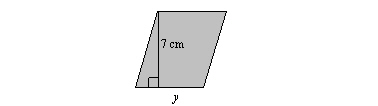a. 4 cm c. 6 cm b. 12 cm d. 14 cm

5.

Perimeter = 12 in.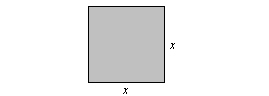a. 4 in. c. 9 in. b. 3 in. d. 6 in.

Find the volume.

6.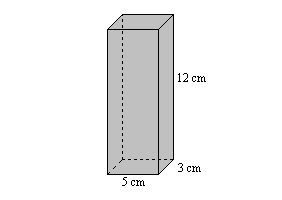a. 60 cmc. 180 cmb. 176 cmd. 15 cmWrite and solve an equation to find the missing dimension of the figure. Check your solution.

7.

Volume = 192 mm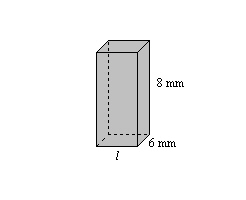a. 48 mm c. 4 mm b. 5 mm d. 15 mm

8.

The fish tank is 80% full. After adding gravel to the bottom, the tank is 85% full. What is the volume of the gravel?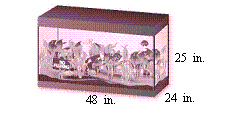a. 3.6 in.c. 4320 in.b. 1440 in.d. 5760 in.Numeric Response

Find the number of cubes it takes to fill the box.

1.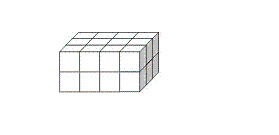2.

How many 1-centimeter cubes are needed to create a cube with a side length of 9 centimeters?